# Description Assignment Convert High Level State Machine Datapath Integrate Corresponding C Q35073062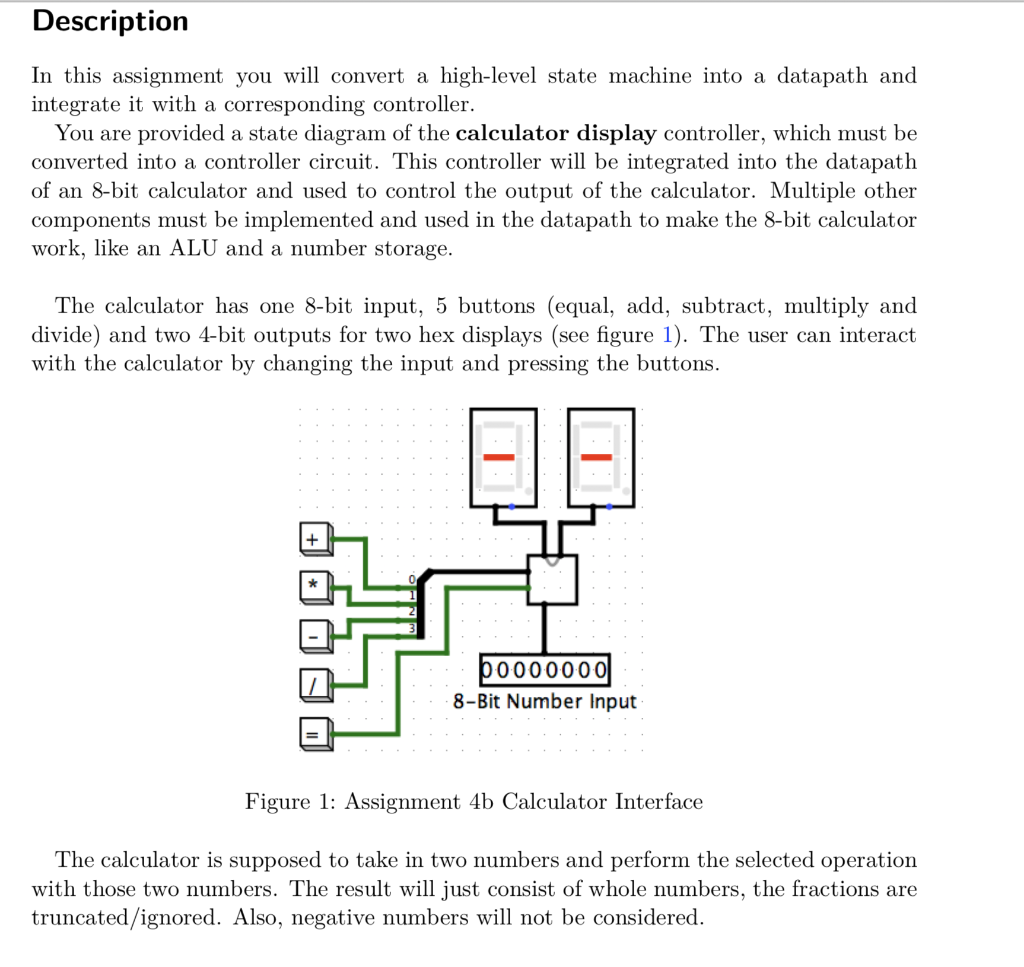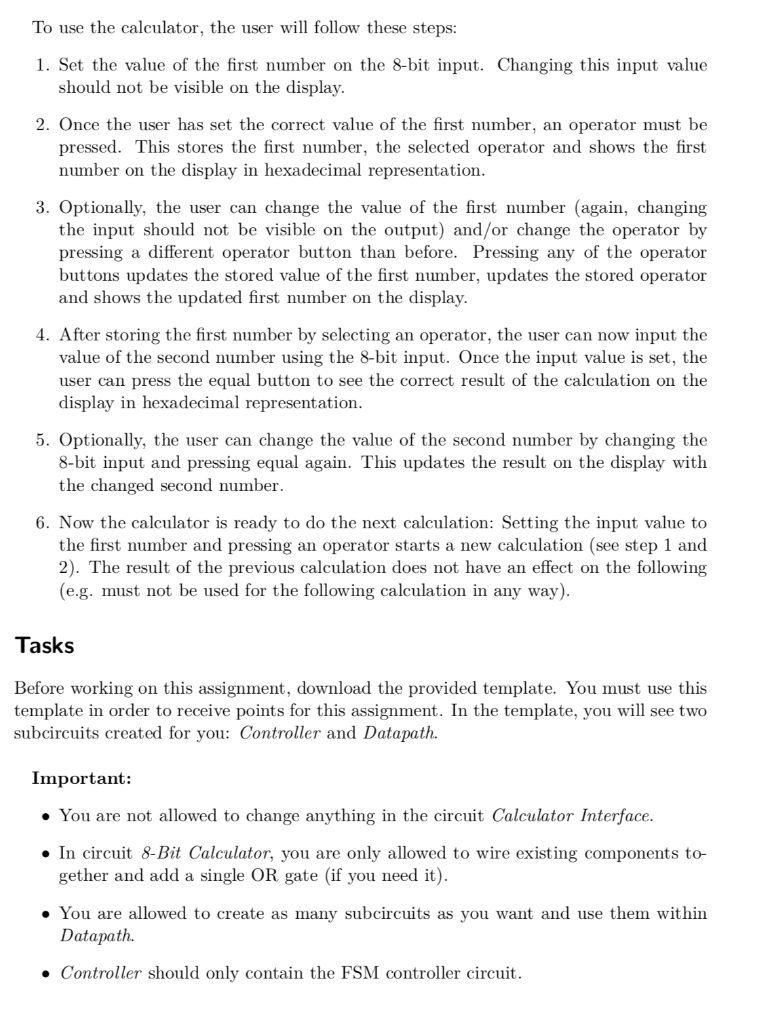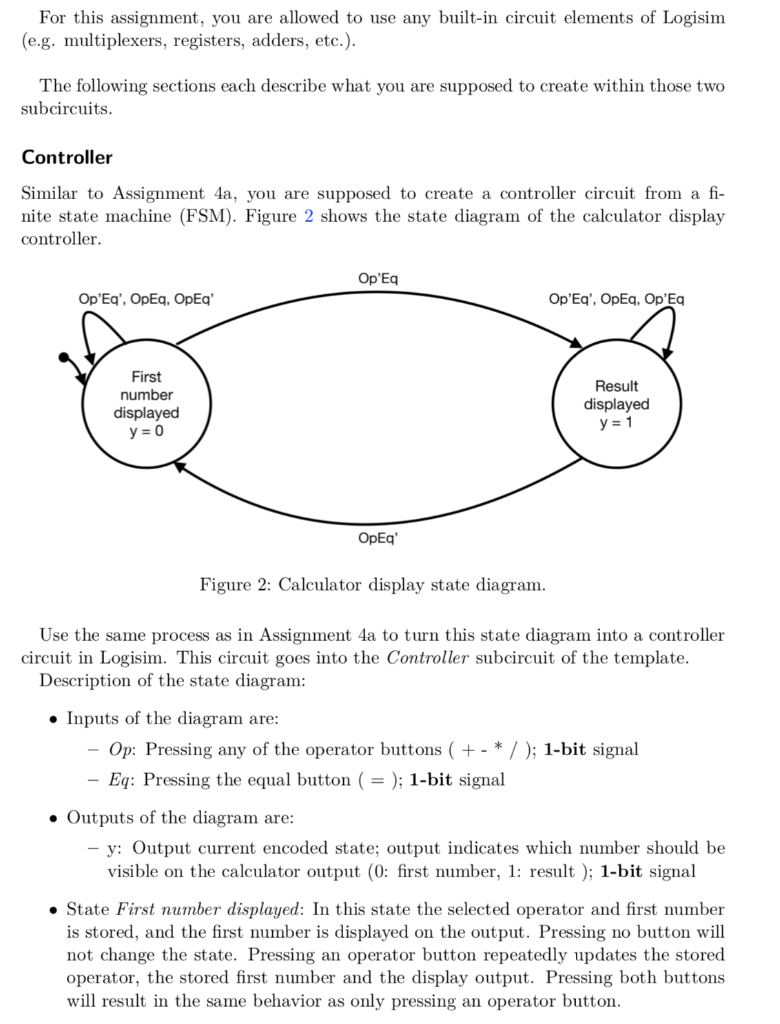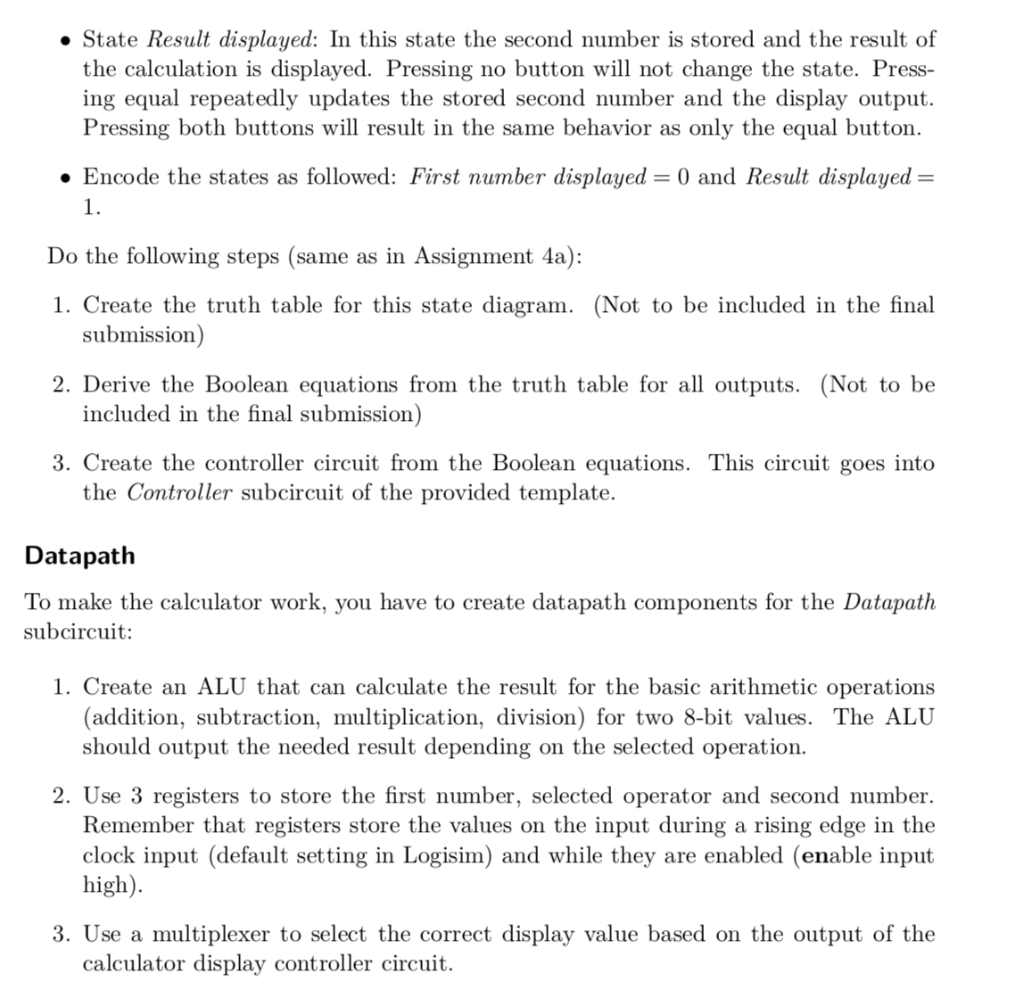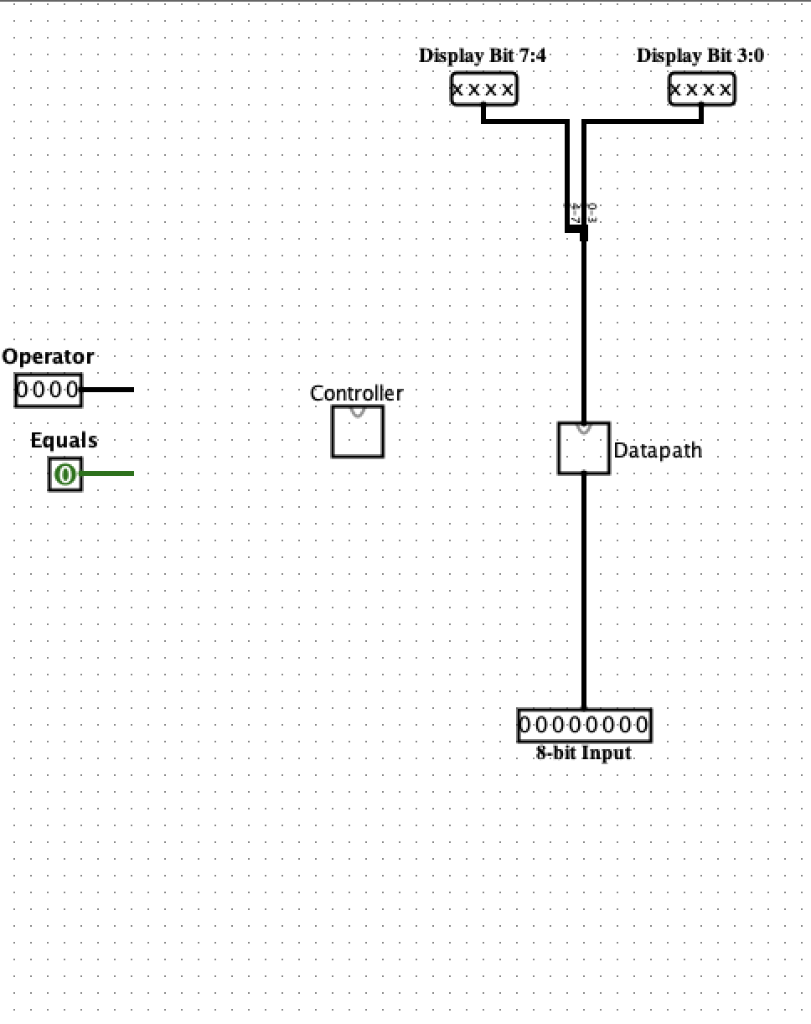8-bit calculator template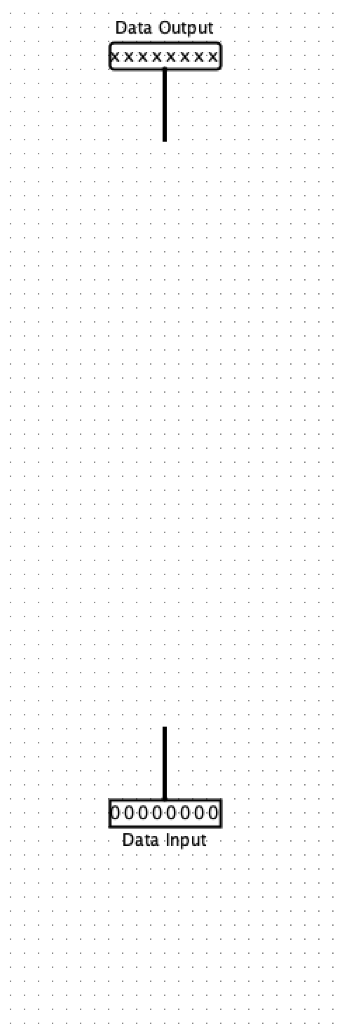datapath templates

Description In this assignment you will convert a high-level state machine into a datapath and integrate it with a corresponding controller. You are provided a state diagram of the calculator display controller, which must be converted into a controller circuit. This controller will be integrated into the datapath of an 8-bit calculator and used to control the output of the calculator. Multiple other components must be implemented and used in the datapath to make the 8-bit calculator work, like an ALU and a number storage. The calculator has one 8-bit input, 5 buttons (equal, add, subtract, multiply and divide) and two 4-bit outputs for two hex displays (see figure 1). The user can interact with the calculator by changing the input and pressing the buttons 8-Bit Number Input Figure 1: Assignment 4b Calculator Interface The calculator is supposed to take in two numbers and perform the selected operation with those two numbers. The result will just consist of whole numbers, the fractions are truncated/ignored. Also, negative numbers will not be considered To use the calculator, the user will follow these steps: 1. Set the value of the first number on the 8-bit input. Changing this input value should not be visible on the display. 2. Once the user has set the correct value of the first number, an operator must be pressed. This stores the first number, the selected operator and shows the first number on the display in hexadecimal representation 3. Optionally, the user can change the value of the first number (again, changing the input should not be visible on the output) and/or change the operator by pressing a different operator button than before. Pressing any of the operator buttons updates the stored value of the first number, updates the stored operator and shows the updated first number on the display 4. After storing the first number by selecting an operator, the user can now input the value of the second number using the 8-bit input. Once the input value is set, the user can press the equal button to see the correct result of the calculation on the display in hexadecimal representation 5. Optionally, the user can change the value of the second number by changing the 8-bit input and pressing equal again. This updates the result on the display with the changed second number. 6. Now the calculator is ready to do the next calculation: Setting the input value to the first number and pressing an operator starts a new calculation (see step 1 and 2). The result of the previous calculation does not have an effect on the following (e.g. must not be used for the following calculation in any way) Tasks Before working on this assignment, download the provided template. You must use this template in order to receive points for this assignment. In the template, you will see two subcircuits created for vou: Controller and Datapath Important: . You are not allowed to change anything in the circuit Calculator Interface » In circuit 8-Bit Calculator, you are only allowed to wire existing components to- gether and add a single OR gate (if you need it) . You are allowed to create as many subcircuits as you want and use them within Datapath. . Controller should only contain the FSM controller circuit For this assignment, you are allowed to use any built-in circuit elements of Logisim (e.g. multiplexers, registers, adders, etc.) The following sections each describe what you are supposed to create within those two subcircuits Controller Similar to Assignment 4a, you are supposed to create a controller circuit from a fi- nite state machine (FSM). Figure 2 shows the state diagram of the calculator display controller Op’Eq Op’Eq’, OpEq, OpEq’ First number displayed Result displayed OpEq’ Figure 2: Calculator display state diagram Use the same process as in Assignment 4a to turn this state diagram into a controller circuit in Logisim. This circuit goes into the Controller subcircuit of the template Description of the state diagram Inputs of the diagram are Op: Pressing any of the operator buttons (+ – * /); 1-bit signal Eq: Pressing the equal button ( = ); 1-bit signal . Outputs of the diagram are y: Output current encoded state; output indicates which number should be visible on the calculator output (0: first number, 1: result); 1-bit signal . State First number displayed: In this state the selected operator and first number is stored, and the first number is displayed on the output. Pressing no button will not change the state. Pressing an operator button repeatedly updates the stored operator, the stored first number and the display output. Pressing both buttons will result in the same behavior as only pressing an operator button . State Result displayed: In this state the second number is stored and the result of the calculation is displayed. Pressing no button will not change the state. Press- ing equal repeatedly updates the stored second number and the display output. Pressing both buttons will result in the same behavior as only the equal button. Encode the states as followed: First number displayed-0 and Result displayed – 1. Do the following steps (same as in Assignment 4a): 1. Create the truth table for this state diagram. (Not to be included in the final submission) included in the final submission) the Controller subcircuit of the provided template. 2. Derive the Boolean equations from the truth table for all outputs. (Not to be 3. Create the controller circuit from the Boolean equations. This circuit goes into Datapath To make the calculator work, you have to create datapath components for the Datapath subcircuit: 1. Create an ALU that can calculate the result for the basic arithmetic operations (addition, subtraction, multiplication, division) for two 8-bit values. The ALU should output the needed result depending on the selected operation. 2. Use 3 registers to store the first number, selected operator and second number. Remember that registers store the values on the input during a rising edge in the clock input (default setting in Logisim) and while they are enabled (enable input high). 3. Use a multiplexer to select the correct display value based on the output of the calculator display controller circuit. Display Bit 7:4 Display Bit 3:0 Operator Controller Equals 0 Datapath 回- 8-bit Input Data Output Data Input Show transcribed image text